SEARCH HOMEMath Central Quandaries & QueriesQuestion from Shanti, a student: how do you graph a step function or piecewise function such as u^2 if u is greater than or equal to -2 and less than 1 2- u if u is greater than or equal to 1 and less than 4Hi Shanti,

I would start by sketching both graphs y = u2 and y = 2 - u making sure that I covered the entire range of the u-values, -2 to 4.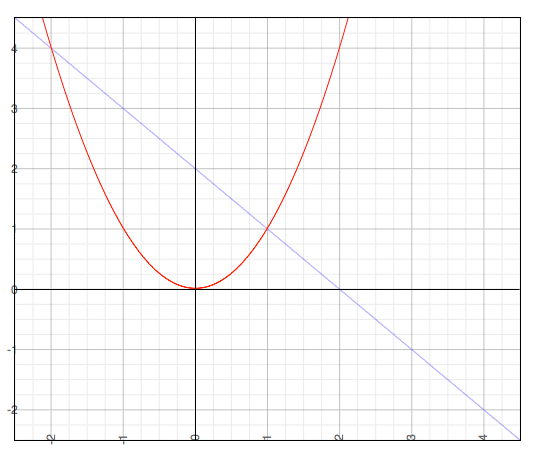y = u2 and y = 2 - u

But you only want pieces of these graphs. The piece of the parabola y = u2 for u between -2 and 1,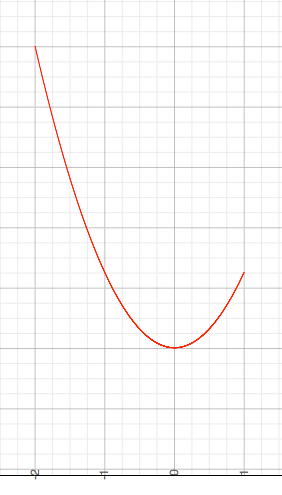and the piece of the line y = 2 - u for u between 1 and 4.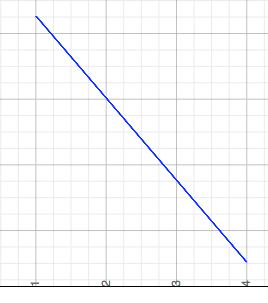Hence removing the unwanted pieces from the graph containing both equations I get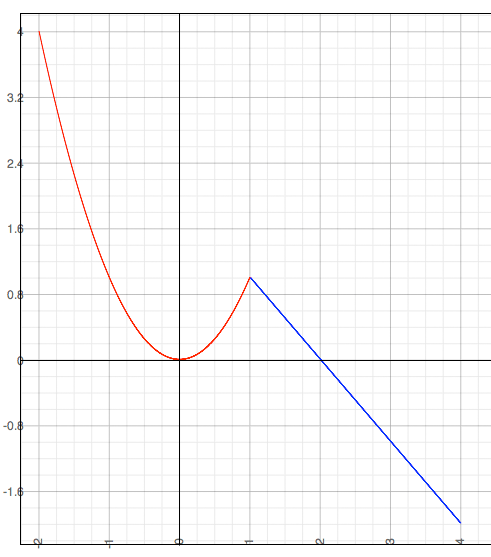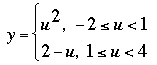I hope this helps,
PennyMath Central is supported by the University of Regina and The Pacific Institute for the Mathematical Sciences.